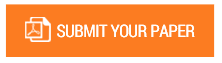New Exact Solution of a Class of Kuramoto Sivashinsky (KS) Equations using Double Reduction Theory

Authors : J. E. Okeke; O.C. Okoli; T. A. Obi; R.N. Ujumad

Volume/Issue : Volume 7 - 2022, Issue 3 - March

DOI : https://doi.org/10.5281/zenodo.6396936

Conservation laws and symmetries of partial differential equations (PDEs) are very useful in finding new methods for reducing PDEs. In this paper, we study the conservation laws and symmetries of a class of a famous fourth-order Kuramoto Sivashinsky (KS) equation. The invariance properties of the conserved vectors with the Lie point symmetry generators are examined using the Double reduction method. With the Double reduction method, the equation is reduced into solvable PDEs or even ordinary differential equations. Some of these reductions yielded some important differential equations that have been investigated by many reseachers. Furthermore, we obtain important and nontrivial solution in terms of generalized Hypergeometric function which possesses significant features in evolution phenomena. Our results not only contributed extra features to the already existing solutions in literature but are also useful in the analysis of wave propagation in plasma, solid state and fluid physics.

Keywords : Lie Symmetries; Conservation Laws; Double Reduction; Exact Solutions

#### CALL FOR PAPERS

Paper Submission Last Date
31 - December - 2023